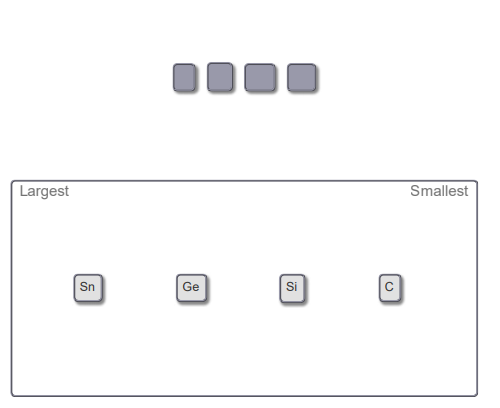# Problem: Rank the following elements in order of decreasing atomic radius.The atomic radius of an element can be predicted based on its periodic properties. Atomic radii increase going down a group in the periodic table, because successively larger valence-shell orbitals are occupied by electrons. Atomic radii generally decrease moving from left to right across a period because the effective nuclear charge increases.

###### Problem Details

Rank the following elements in order of decreasing atomic radius.The atomic radius of an element can be predicted based on its periodic properties. Atomic radii increase going down a group in the periodic table, because successively larger valence-shell orbitals are occupied by electrons. Atomic radii generally decrease moving from left to right across a period because the effective nuclear charge increases.

Frequently Asked Questions

What scientific concept do you need to know in order to solve this problem?

Our tutors have indicated that to solve this problem you will need to apply the Periodic Trend: Atomic Radius concept. You can view video lessons to learn Periodic Trend: Atomic Radius. Or if you need more Periodic Trend: Atomic Radius practice, you can also practice Periodic Trend: Atomic Radius practice problems.